# Multiplication and Division of Whole Numbers

## Objective

Multiply two-digit numbers by two-digit numbers.

## Common Core Standards

### Core Standards

?

• 5.NBT.B.5 — Fluently multiply multi-digit whole numbers using the standard algorithm.

?

• 4.NBT.B.4

• 4.NBT.B.5

• 5.NBT.A.1

• 5.NBT.A.2

## Criteria for Success

?

1. Compute two-digit by two-digit products using the area model, the partial products algorithm, and the standard algorithm.
2. Relate the area model, the partial products algorithm, and the standard algorithm.
3. Round to estimate two-digit by two-digit products.

## Tips for Teachers

?

Students have seen the area model, the partial products algorithm, and the standard algorithm strategies with two-digit by two-digit multiplication in Grade 4. However, because two-digit by two-digit multiplication is both more complex than all of the cases students saw in Lesson 5 and more closely related to the new cases they’ll see in Grade 5, a whole day has been dedicated to this case to solidify the standard algorithm with this case before moving on to more cognitively complex and new cases in later lessons.

#### Fishtank Plus

• Problem Set
• Student Handout Editor
• Vocabulary Package

?

### Problem 1

1. Here are two ways to find the area of a rectangle that is 23 units by 31 units.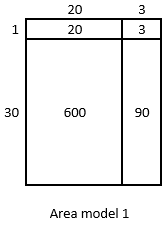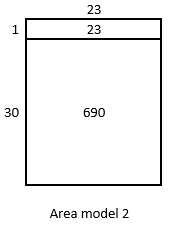1. What do the area models have in common? How are they alike?
2. How are they different?
3. If you were to find the area of a rectangle that is 37 units by 49 units, which of the three ways of decomposing the rectangle would you use? Why?

2. You might be familiar with different ways to write multiplication calculations. Here are two ways to calculate 23 x 31.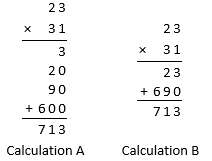a. In Calculation A, how are each of the partial products obtained? For instance, where does the 3 come from?

b. In Calculation B, how are the 23 and 690 obtained?

c. Look at the area models in #1. Which area model corresponds to Calculation A? Which one corresponds to Calculation B?

d. How are the partial products in Calculation A and the 23 and 690 in Calculation B related to the numbers in the area models?

#### References

Open Up Resources Grade 6, Unit 5, Lesson 7, Teacher Version7.2: Connecting Area Diagrams to Calculations with Whole Numbers

Modified by The Match Foundation, Inc.

### Problem 2

1. Label the area model to represent 37 x 49 and to find that product.

1. Decompose each number into its base-ten units (tens, ones, etc.) and write them in the boxes on each side of the rectangle.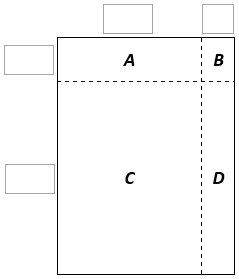1. Label regions A, B, C, and D with their areas. Show your reasoning.
2. Find the product that the area model represents. Show your reasoning.

2. Here are two ways to calculate 37 x 49. Each number with a box gives the area of one or more regions in the area model.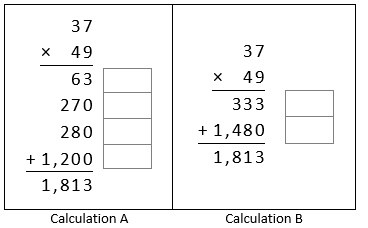1. In the boxes next to each number, write the letter(s) of the corresponding region(s).
2. In Calculation B, which two numbers are being multipled to obtain 333? Which two are being multiplied to obtain 1,480?

#### References

Open Up Resources Grade 6, Unit 5, Lesson 7, Teacher Version7.4: Using the Partial Products Method

Modified by The Match Foundation, Inc.

### Problem 3

Estimate the following products. Then solve using the standard algorithm. If you get stuck, use an area model and/or the partial products algorithm to help.

1. 73 × 64
2. 48 × 67

#### References

Open Up Resources Grade 6, Unit 5, Lesson 7, Teacher Version7.4: Using the Partial Products Method

Modified by The Match Foundation, Inc.

## Discussion of Problem Set

?

• Look at #2e and #2f. How did you assess reasonableness for both problems? If you round both factors to the nearest ten in both problems, you get the same estimate. Why does it make sense that the actual product in #2e is less than the estimate and the actual product in #3f is larger than the estimate?
• Look at #3. How did you determine which expression was equal to 36 × 94? How is this related to the area model? To the standard algorithm?
• Look at #4. How did you solve for the values of B and C?
• How did you determine where to place the digits in #7?
• Look at #8. What was the biggest product you were able to make? The smallest? How did you find those values?

?

### Problem 1

Solve. Show or explain your work.

1.   21 x 23
2.   78 x 42

#### References

EngageNY Mathematics Grade 5 Mathematics > Module 2 > Topic B > Lesson 5Exit Ticket

Grade 5 Mathematics > Module 2 > Topic B > Lesson 5 of the New York State Common Core Mathematics Curriculum from EngageNY and Great Minds. © 2015 Great Minds. Licensed by EngageNY of the New York State Education Department under the CC BY-NC-SA 3.0 US license. Accessed Dec. 2, 2016, 5:15 p.m..

Modified by The Match Foundation, Inc.
EngageNY Mathematics Grade 5 Mathematics > Module 2 > Topic B > Lesson 6Exit Ticket

Grade 5 Mathematics > Module 2 > Topic B > Lesson 6 of the New York State Common Core Mathematics Curriculum from EngageNY and Great Minds. © 2015 Great Minds. Licensed by EngageNY of the New York State Education Department under the CC BY-NC-SA 3.0 US license. Accessed Dec. 2, 2016, 5:15 p.m..

Modified by The Match Foundation, Inc.

### Problem 2

Ashley drew an area model to find the value of 85 x 17.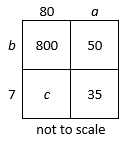• Determine the number that each letter in the model represents and explain each of your answers.
• Write the product for 85 x 17.

?